# 羽尾

Feathertail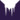杀无尽部落 (Q640)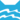河族 (Q630)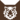虎族 (Q645)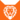狮族 (Q646)河族 武士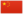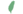羽崽 Featherkit  小羽
 羽爪 Featherpaw  羽掌
 羽尾 Feathertail  羽尾

Q3459：河族母猫，原雷族猫，自《疑云重重》登场的角色

## 细节

### 外貌

• 她是一只身形苗条、天:3章蓝色眼睛:猫物表的银色:308虎斑:43母猫。:猫物表她的皮毛柔软似云、:197面部宽阔，:189有一条似烟:197的蓬松尾巴。:64
• 她的外貌和母亲银溪极为相似。:308

## 登场书目

• 疑云重重 (Q60)[配角][首次登场🐱]
• 风起云涌 (Q65)[配角]
• 险路惊魂 (Q66)[对话提及]
• 力挽狂澜 (Q67)[配角]
• 午夜追踪 (Q139)[主要角色]
• 新月危机 (Q140)[主要角色][视角人物][死亡时刻✝]
• 重现家园 (Q141)[配角]
• 星光指路 (Q142)[配角]
• 黄昏战争 (Q143)[配角]
• 日落和平 (Q144)[配角]
• 预视力量 (Q152)[对话提及]
• 驱逐之战 (Q154)[配角]
• 暗夜长影 (Q156)[对话提及]
• 第四学徒 (Q158)[配角]
• 战声渐近 (Q159)[对话提及]
• 武士归来 (Q162)[间接提及]
• 群星之战 (Q163)[对话提及]
• 烈焰焚河 (Q174)[间接提及]
• 风暴来袭 (Q175)[间接提及]
• 迷雾明光 (Q4621)[配角]
• 火星的探索 (Q176)[配角]
• 钩星的承诺 (Q179)[配角]
• 黑莓星的风暴 (Q182)[对话提及]
• 鸦羽的拷问 (Q186)[配角]
• 松鼠飞的希冀 (Q3561)[对话提及]
• 灰条的誓言 (Q4057)[仅于幻象]
• 豹星的荣光 (Q4426)[配角]
• 一星的告解 (Q4826)[对话提及]
• 雾星的征兆 (Q188)[配角]
• 叶池的祈愿 (Q191)[配角]
• 枫荫的报复 (Q193)[间接提及]
• 蛾翅的秘密 (Q3628)[对话提及]
• 黑脚的审判 (Q4336)[仅于回忆]
• 河族阴云 (Q4311)[主人公][主要角色][视角人物]
• 武士失踪 (Q207)[配角]
• 返回族群 (Q212)[配角]
• 族群的秘密 (Q199)[追溯修订]
• 族群的猫 (Q200)[配角]
• 终极指南 (Q204)[配角]

## 参考文献

这篇文章基于CC BY-SA 3.0许可使用了猫武士维基（英语）Feathertail一文中的部分内容。
1. 《新月危机》第二十三章 (Q969)
2. 《疑云重重》第二十一章 (Q820)
3. 风起云涌 (Q65)
4. 《险路惊魂》第二十二章
5. 雾星的征兆
6. 午夜追踪
7. 疑云重重
8. 迷雾明光
9. 预视力量
10. 呼唤野性
11. 蓝星的预言
12. 钩星的承诺
13. Su Susann (2016-09-30). 站内截图. Facebook . 原页面 归档于 2017-10-31. “As I said, Adderfang is a descandant of Spottedpelt and Pinestar is, trough the blood of his father, related to Gorseclaw.”
14. 重现家园